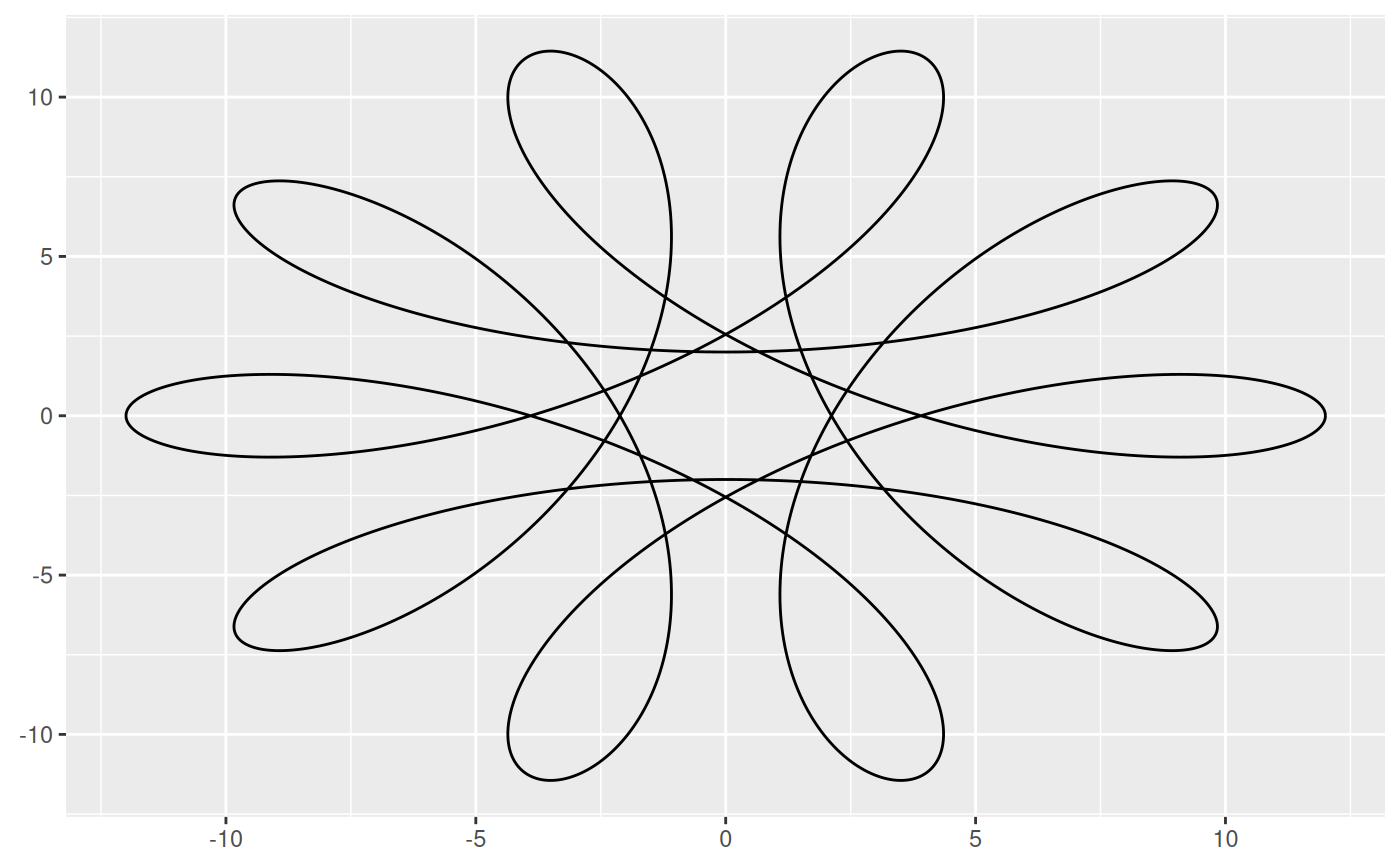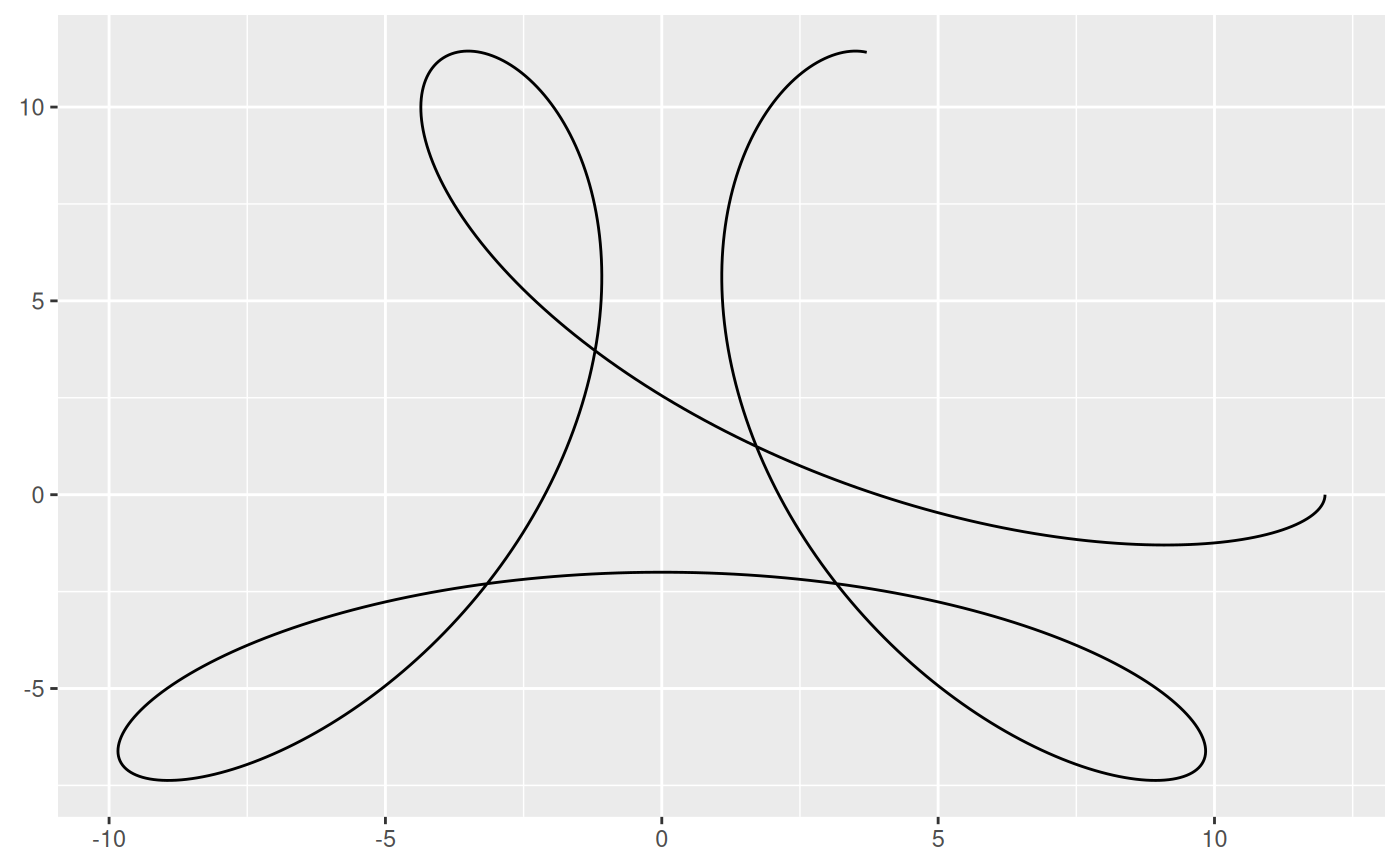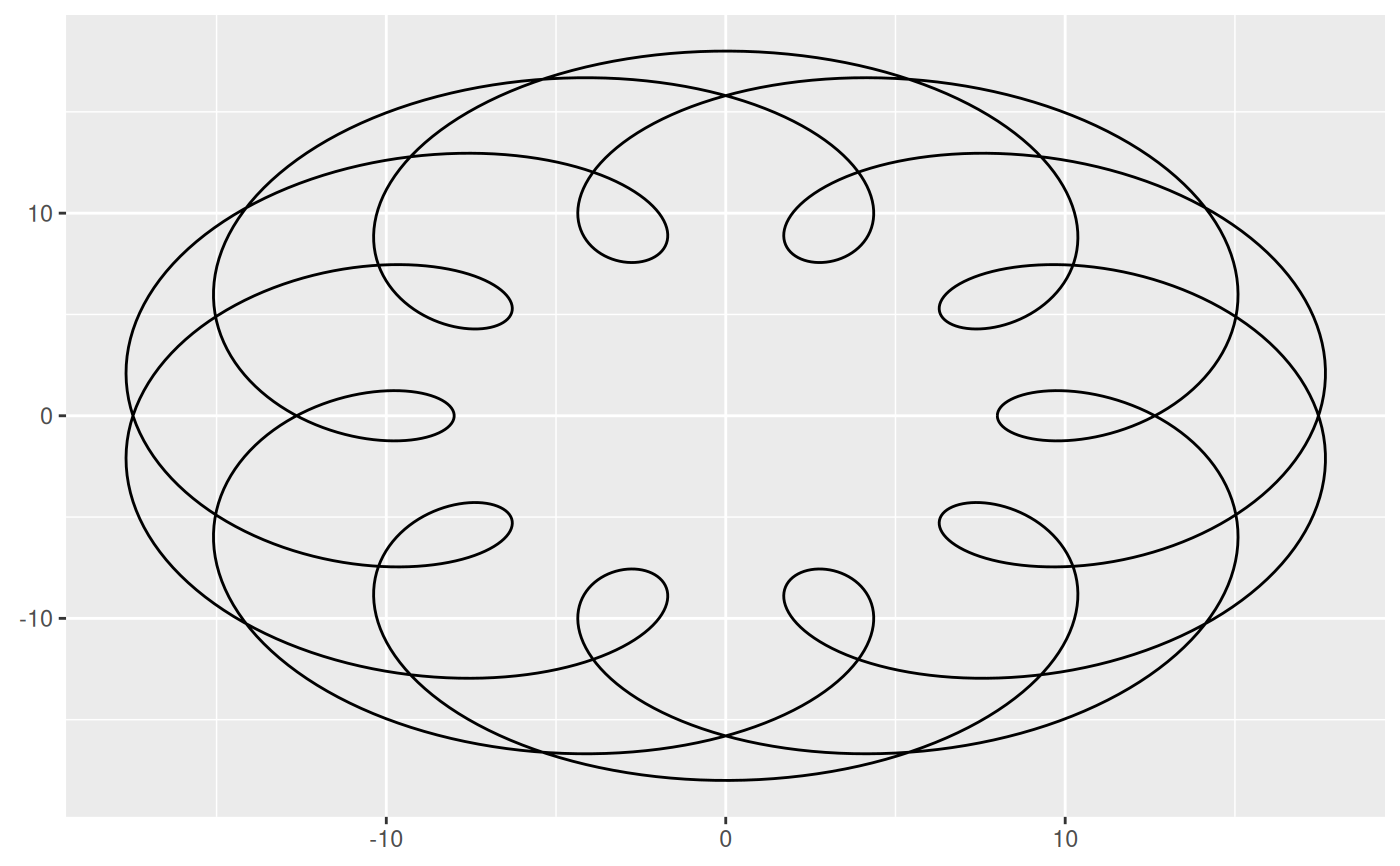This, rather pointless, geom allows you to draw spirograms, as known from the popular drawing toy where lines were traced by inserting a pencil into a hole in a small gear that would then trace around inside another gear. The potential practicality of this geom is slim and it excists mainly for fun and art.

stat_spiro(
mapping = NULL,
data = NULL,
geom = "path",
position = "identity",
na.rm = FALSE,
n = 500,
revolutions = NULL,
show.legend = NA,
inherit.aes = TRUE,
...
)

geom_spiro(
mapping = NULL,
data = NULL,
stat = "spiro",
position = "identity",
arrow = NULL,
n = 500,
lineend = "butt",
na.rm = FALSE,
show.legend = NA,
inherit.aes = TRUE,
...
)

## Arguments

mapping

Set of aesthetic mappings created by aes(). If specified and inherit.aes = TRUE (the default), it is combined with the default mapping at the top level of the plot. You must supply mapping if there is no plot mapping.

data

The data to be displayed in this layer. There are three options:

If NULL, the default, the data is inherited from the plot data as specified in the call to ggplot().

A data.frame, or other object, will override the plot data. All objects will be fortified to produce a data frame. See fortify() for which variables will be created.

A function will be called with a single argument, the plot data. The return value must be a data.frame, and will be used as the layer data. A function can be created from a formula (e.g. ~ head(.x, 10)).

geom

The geometric object to use to display the data, either as a ggproto Geom subclass or as a string naming the geom stripped of the geom_ prefix (e.g. "point" rather than "geom_point")

position

Position adjustment, either as a string naming the adjustment (e.g. "jitter" to use position_jitter), or the result of a call to a position adjustment function. Use the latter if you need to change the settings of the adjustment.

na.rm

If FALSE, the default, missing values are removed with a warning. If TRUE, missing values are silently removed.

n

The number of points that should be used to draw a fully closed spirogram. If revolutions < 1 the actual number of points will be less than this.

revolutions

The number of times the inner gear should revolve around inside the outer gear. If NULL the number of revolutions to reach the starting position is calculated and used.

show.legend

logical. Should this layer be included in the legends? NA, the default, includes if any aesthetics are mapped. FALSE never includes, and TRUE always includes. It can also be a named logical vector to finely select the aesthetics to display.

inherit.aes

If FALSE, overrides the default aesthetics, rather than combining with them. This is most useful for helper functions that define both data and aesthetics and shouldn't inherit behaviour from the default plot specification, e.g. borders().

...

Other arguments passed on to layer(). These are often aesthetics, used to set an aesthetic to a fixed value, like colour = "red" or size = 3. They may also be parameters to the paired geom/stat.

stat

The statistical transformation to use on the data for this layer, either as a ggproto Geom subclass or as a string naming the stat stripped of the stat_ prefix (e.g. "count" rather than "stat_count")

arrow

Arrow specification, as created by grid::arrow().

lineend

Line end style (round, butt, square).

## Aesthetics

stat_spiro and geom_spiro understand the following aesthetics (required aesthetics are in bold):

• R

• r

• d

• x0

• y0

• outer

• color

• size

• linetype

• alpha

## Computed variables

x, y

The coordinates for the path describing the spirogram

index

The progression along the spirogram mapped between 0 and 1

## Examples

# Basic usage
ggplot() +
geom_spiro(aes(R = 10, r = 3, d = 5))# Only draw a portion
ggplot() +
geom_spiro(aes(R = 10, r = 3, d = 5), revolutions = 1.2)# Let the inner gear circle the outside of the outer gear
ggplot() +
geom_spiro(aes(R = 10, r = 3, d = 5, outer = TRUE))